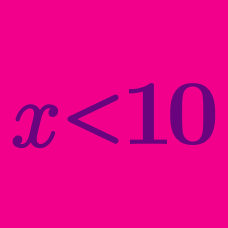Algebra

# Multi-Step Linear Inequalities

If $x$ is an integer that satisfies the inequality $12 x \leq 8x+ 128$, what is the largest possible value of $x$?

What is the maximum integer $x$ that satisfies the inequality $3(x+1)-30 < x+15?$

Solve $\displaystyle \frac{x-3}{3}>2x+14.$

Solve $\frac{\frac{x}{5}}{6} > 1.$

What is the maximum value of $x$ that satisfies $\frac{x}{4} + 7 \leq 15$?

×